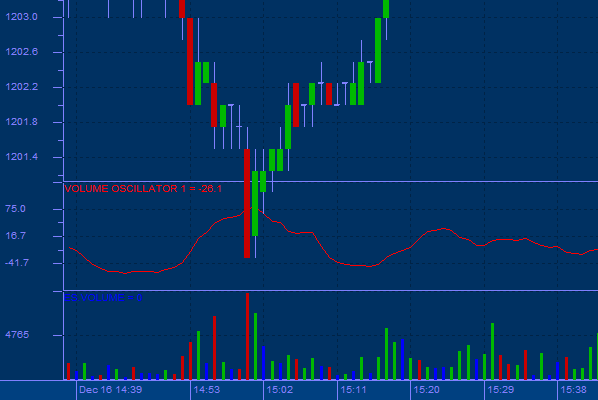top of page## Volume Oscillator

The Trade Volume Oscillator displays the difference between two moving averages of a volume. The difference between the moving averages can be expressed in either points or percentage.

Usage:

You can use the difference between two moving averages of volume to determine if the overall volume trend is increasing or decreasing. When the Volume Oscillator rises above zero, it signifies that the shorter-term volume moving average has risen above the longer-term volume moving average, and thus, that the short-term volume trend is higher (i.e., more volume) than the longer-term volume trend.bottom of page